All news

# Give us your best (Mathematica) one-liner

Here’s another guest post from Allison Taylor at Wolfram, which is part of a series that will help smooth your introduction to Mathematica. Thanks Allison: over to you!

At our 2010 and 2011 Wolfram Technology Conferences, attendees were challenged to come up with their best one-liners—pieces of code written in less than one line, or under 140 characters. The outputs were then judged by their complexity, usefulness, and general cool-factor. The submissions turned out to be quite diverse, thought provoking, and most of all, pretty nifty illustrations of the efficiency of the Wolfram Language.

So it only seemed fitting to tailor some of these small bundles of powerful code to the equally small-but-mighty Raspberry Pi. (To run these, you must first open the front-end version of Mathematica on your Pi by clicking the Mathematica icon.)

## Echidnahedron (AKA, the Mathematica Spikey), by Radko Kriz

We couldn’t help but start off with teaching you how to make a cool, geometric logo similar to the one that has been representing Mathematica and Wolfram Research for over 25 years! The echidnahedron is a “stellation” of the icosahedron—basically, it takes the faces of the icosahedron and turns them into infinitely extending spikes. Or Spikey, as we like to call it.

```Graphics3D[{Opacity[.8], Glow[RGBColor[1,0,0]], EdgeForm[White], Lighting ->
None, PolyhedronData["Echidnahedron", "Faces"]}]
```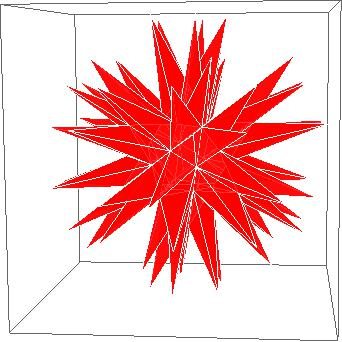## The Ulam Spiral, by Dave Lawrence

Along a similar mathy theme, the Ulam Spiral is a beautiful way to visualize prime numbers. The spiral is contructed on an integer coordinate plane, starting at (0,0) and spiraling outward through every point that is prime.

```s = {Re@#, Im@#} & /@
Fold[Join[#1, Last@#1 + I^#2 Range@#2/2] &, {0},
Range@140]; ListPlot[s, Joined -> True,
Epilog -> {Point /@ s[[Prime@Range@PrimePi@Length@s]]}]
```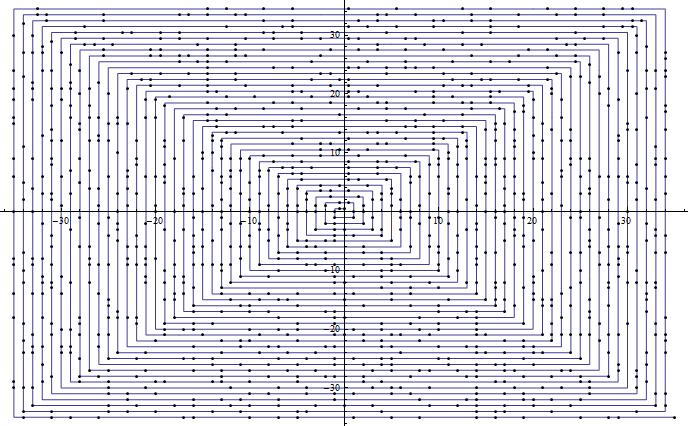Not the biggest bang Stanislaw Ulam ever made, but neat nonetheless

## Test If an Integer Is a Prime Number, by Sascha Kratky

And while we’re on the subject of prime numbers, there are tons of math and computation examples where this little gem of code will likely come in handy. Run this function with any integer, and it will return True if it is prime and False if it is not.

```RegExPrimeQ[n_Integer] := !
StringMatchQ[StringJoin[Table["1", {Abs[n]}]],
RegularExpression["^1?\$|^(11+?)\\1+\$"]] RegExPrimeQ
```

## Make Your Own Borg Cube, by Alex Hirsbrunner

You know you’ve always wanted to make one. By tiling a bunch of randomly generated cubes of random sizes and colors, this code will create your very own one-of-a-kind Borg cube to assimilate all your friends into the hive mind.

```p := Random[];
r = RotateLeft;
Graphics3D[
Table[{RGBColor[.2, p, .2],
Cuboid[l =
r[{z, p, p}, a], l +
r[p/
8 {Sign[z - .5], p, p}, a]]}, \ {z, {0, 1}}, {a, 0, 2}, {6!}]]
```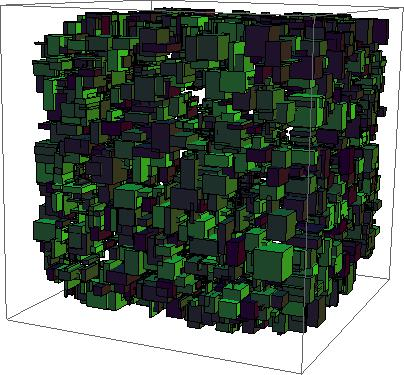## Fractals, by Stephan Leibbrandt

Make a “heart-shaped box of wires and springs” with this nifty line of code!

```Image@Compile[{},
Block[{i, x, p},
Table[i = 0; x = 0. I; p = r + I c;
While[Abs@x
Tanh[Power[i/9^3, (7)^-1]], {c, -1, 1, .01}, {r, -2, 1,.01}]]][]
```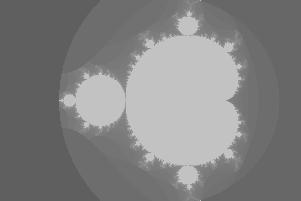## Rubber Duck, by Paul Abbott

And we’ll leave you with this cute little creation, made using a series of spherical functions, because it’s just adorable, isn’t it?

```SphericalPlot3D[
Log[\[Theta]] + Sin[\[CurlyPhi]], {\[Theta], 0,
2 \[Pi]}, {\[CurlyPhi], 0, 2 \[Pi]}, Boxed -> False, Axes -> False,
PlotStyle -> Yellow, Mesh -> None, PlotPoints -> 30]
```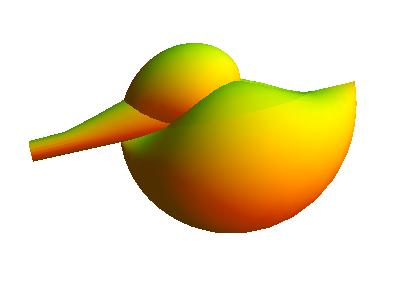Inspired? Show us what Raspberry Pi oriented one-liners you can come up with!

The equation that says ‘Hi’

Plot3D[Exp[-x^2 – 0.5*y^2]*Cos[4 x] +
Exp[-3 ((x + 0.5)^2 + 0.5 y^2)], {x, -3, 3}, {y, -5, 5},
PlotRange -> {-0.001, 0.001}, PlotPoints -> 40]

Kudos to you Michael – my first thought when I saw this post was to wonder if anyone had come up with a neat Hello World in a one liner.

Kudos.

*Desperately searches for a Mathematica related pun*

The Raspberry Pi Guy

Mathematica walked into a bar graph…perhaps it was a sine.

Well, that just sums it up……

I’ve got nothing more to add…

Maths puns can be so divisive!

you’ve gone on a bit of a tangent. maybe we need someone to cosine your sectioning papers

Not a Mathematica joke, but my favourite maths joke is:

Q. What does the B in Benoit B Mandelbrot stand for?

A. Benoit B Mandelbrot.

I love that bit!

There are students in our school who are heavily into art, but “hate” math(s) without being aware of what can be produced beyond the tired old plot of quadratic equations in 2-D that is pretty much as far as most students can stand to produce. Now I have a flute named Mathematica with which to herd those cats, or some such other completely broken metaphor. We have an open house tonight where I’ll be showing off our recently-acquired bevy of boards de Pi and I’ll be setting up a number of them with some very tantalizing Mathematica plots. I’ll challenge the students, parents, and other teachers to come up with some of their own one-liners.

Thanks again to Wolfram for making this available to all of us in the Pi and educational communities at no charge, making the price of the Pi an even more ridiculously incredible value than it already is for just the board.

Jim, that sounds great! We’d love to hear how it goes tonight.

It’s a “heart-shaped box of springs and wires” ackchewerly ;-)

Cool stuff though :-)

a small flower

RevolutionPlot3D[Tanh[2 r], {r, 0, 1.2},
RegionFunction -> Function[{x, y}, (x^2 + y^2)^3 None, ColorFunction -> “RoseColors”, Boxed -> False,
Axes -> False, MaxRecursion -> 5]

Is there a missing square bracket?

Sorry, there is a part missing in the syntax. This is the correct one.

RevolutionPlot3D[Tanh[2 r], {r, 0, 1.2},
RegionFunction -> Function[{x, y}, (x^2 + y^2)^3 None, ColorFunction -> “RoseColors”, Boxed -> False,
Axes -> False, MaxRecursion -> 5]

RevolutionPlot3D[Tanh[2 r], {r, 0, 1.2}, RegionFunction -> Function[{x, y}, (x^2 + y^2)^3 None, ColorFunction -> “RoseColors”, Boxed -> False, Axes -> False, MaxRecursion -> 5]

for some reason it is not showing less than (” None,

An atom “walks” over to his friend and says,’Whoa! I think you lost your electron.” He replies,”No I didn’t.” “Are you sure??” “Positive”

hehehe

Did you make that up?

If you did it is awesome :)

A neutron walks into a bar and orders a beer. Bartender says ‘No charge!’

√69 = 8.something

How do you install mathematica?

Mathematica and Wolfram Language are included in the latest Noobs distribution. After downloading and installing it they show up on the desktopm.

Some information on alternative ways of installing them is available on the Wolfram Community website
http://community.wolfram.com/groups/-/m/t/157340?p_p_auth=U6GWwn28

I don’t have latest and I am to lazy to reinstall everything :)

There’s also this blog post
http://www.raspberrypi.org/archives/5282

Shades of APL! The return of the incomprehensible one-liner.

The APL conferences were the geekiest I ever attended. Someone would walk in wearing a tee shirt with two or three inscrutable characters printed on it and half the room would fall on the floor laughing.

Mathematics One-Liners?
There are 10 types of people in this world: those who understand binary, and those who don’t.

You didn’t say Mathematics? Oh…

that ruuber duck looks so fat , ratio of circumference to diameter must be 4!

What? Checking for primality with regular expressions on a unary representation of the number? Yuck.
How about a plain old PrimeQ[n]?

I tried to draw a volumetric Raspberry Pi logo with 140 characters or less, but I couldn’t manage it. This is my best shot, with 162 characters:
```Graphics3D[{Red, Sphere /@ PolyhedronData["Icosidodecahedron"][[1, 1]], Green, GeometricTransformation[ Cylinder[], {{# {1, 0, -1}, {0, 0, .3}, {1, 1, -1}}, {-# .1, 0, 1.5}}] & /@ {-1, 1}}]```

And here is the result, it was lost from my first post: http://i.imgur.com/v4kuEUT.png

Hey Martin,

That’s a really cool idea! I modified your code to just 140 characters and added a bit of coolness to it (it’s upside down but a minus would take the characters over the limit):

s = Sphere; t = .3; p = PolyhedronData; Manipulate[
Graphics3D@{Red, s@p[i][[1, 1]], Green,
Rotate[Scale[s[], {t, 1, 2}, {0, 0, 3}], #, {1, 0, 0}] & /@ {- t,
t}}, {i, p[]}]

I tried the Borg Cube, but it complains that ‘RotateLeft’ needs a parameter – has anyone else tried & corrected this?

Hm, maybe it doesn’t like r=RotateLeft;

Try:

p := Random[];
Graphics3D[
Table[{RGBColor[.2, p, .2],
Cuboid[l = RotateLeft[{z, p, p}, a],
l + RotateLeft[p/8 {Sign[z – .5], p, p}, a]]}, {z, {0, 1}}, {a, 0,
2}, {6!}]]

I had exactly the same problem. I even tried different values – no success :-(

Looking at the one-liners page here it would appear the the fractals example is missing a fair bit of code above. The correct code for the fractal appear like it should be:

```Image@Compile[{}, Block[{i, x, p}, Table[i = 0; x = 0. I; p = r + I c; While[Abs@x <= (2)^-1 && i < 9^3, x = x^2 + p; ++i]; Tanh[Power[i/9^3, (7)^-1]], {c, -1, 1, .01}, {r, -2, 1, .01}]]][]```

However this still does not work correctly. Must just be a tiny little error, but my brain is too fried to work it out right now!

Wolfram is unsuited to an OPEN-source project like rPi:
1= bloated code-base;
2= semi-secretive tutorial-docos, where a few words of Walt-Disney
style text, is fetched for each massive [and repeated] commercial bloat.
3= docos/tutorials are LOCKED to Wolfram, requiring an always-on inet
connection, and willingness to have outsiders hold-your-hand and